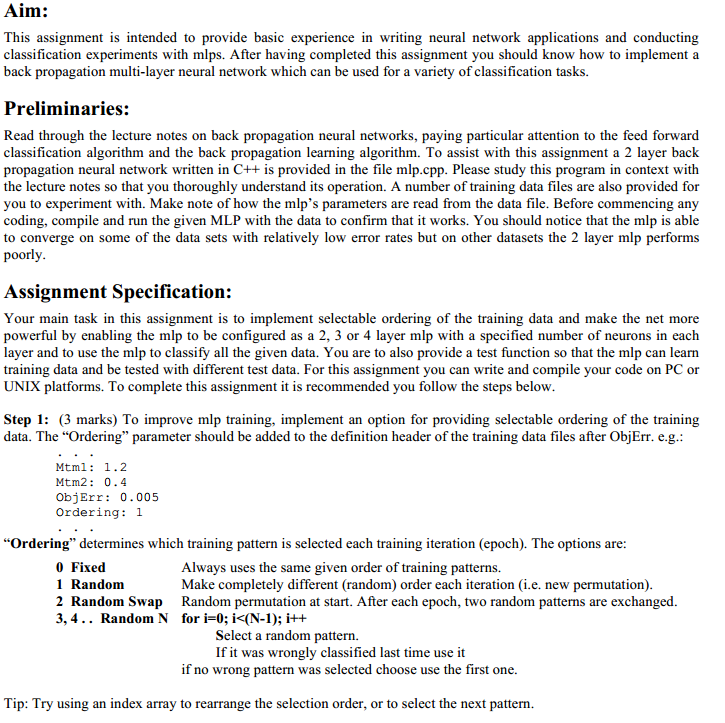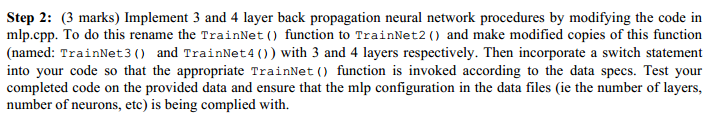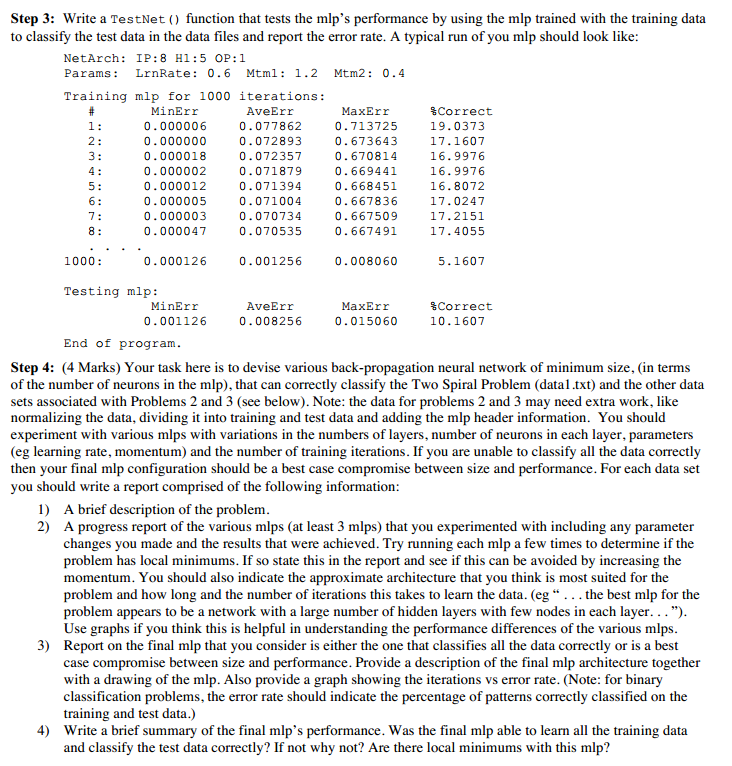# We Helped With This Programming Homework: Have A Similar One?

SOLVEDCategory Programming Other Graduate Solved Computer Science Homework Help

## Assignment ImageAim: This assignment is intended to provide basic experience in writing neural network applications and conducting classification experiments with mlps. After having completed this assignment you should know how to implement a back propagation multi-layer neural network which can be used for a variety of classification tasks. Preliminaries: Read through the lecture notes on back propagation neural networks, paying particular attention to the feed forward classification algorithm and the back propagation learning algorithm. To assist with this assignment a 2 layer back propagation neural network written in C++ is provided in the file mlp.cpp. Please study this program in context with the lecture notes so that you thoroughly understand its operation. A number of training data files are also provided for you to experiment with. Make note of how the mlp's parameters are read from the data file. Before commencing any coding, compile and run the given MLP with the data to confirm that it works. You should notice that the mlp is able to converge on some of the data sets with relatively low error rates but on other datasets the 2 layer mlp performs poorly. Assignment Specification: Your main task in this assignment is to implement selectable ordering of the training data and make the net more powerful by enabling the mlp to be configured as a 2, 3 or 4 layer mlp with a specified number of neurons in each layer and to use the mlp to classify all the given data. You are to also provide a test function so that the mlp can learn training data and be tested with different test data. For this assignment you can write and compile your code on PC or UNIX platforms. To complete this assignment it is recommended you follow the steps below. Step 1: (3 marks) To improve mlp training, implement an option for providing selectable ordering of the training data. The "Ordering" parameter should be added to the definition header of the training data files after ObjErr. e.g.: Mtm1: 1.2 Mtm2: 0.4 ObjErr: 0.005 Ordering: 1 "Ordering" determines which training pattern is selected each training iteration (epoch). The options are: Always uses the same given order of training patterns. Make completely different (random) order each iteration (i.e. new permutation). Random permutation at start. After each epoch, two random patterns are exchanged. for i=0; i<(N-1); i++ Select a random pattern. If it was wrongly classified last time use it if no wrong pattern was selected choose use the first one. Tip: Try using an index array to rearrange the selection order, or to select the next pattern. 0 Fixed 1 Random 2 Random Swap 3,4.. Random N

## Assignment ImageStep 2: (3 marks) Implement 3 and 4 layer back propagation neural network procedures by modifying the code in mlp.cpp. To do this rename the TrainNet() function to TrainNet2 () and make modified copies of this function (named: TrainNet3 () and TrainNet 4 ()) with 3 and 4 layers respectively. Then incorporate a switch statement into your code so that the appropriate TrainNet () function is invoked according to the data specs. Test your completed code on the provided data and ensure that the mlp configuration in the data files (ie the number of layers, number of neurons, etc) is being complied with.

## Assignment ImageStep 3: Write a TestNet () function that tests the mlp's performance by using the mlp trained with the training data to classify the test data in the data files and report the error rate. A typical run of you mlp should look like: NetArch: IP:8 H1:5 OP:1 Params: LrnRate: 0.6 Mtml: 1.2 Mtm2: 0.4 Training mlp for 1000 iterations: # MinErr 1: 2: 3: 4: 5: 6: 7: 8: 1000: 0.000006 0.000000 0.000018 0.000002 0.000012 0.000005 0.000003 0.00004 0.000126 Testing mlp: MinErr 0.001126 AveErr 0.077862 0.072893 0.072357 0.071879 0.071394 0.071004 0.070734 0.070535 0.001256 AveErr 0.008256 MaxErr 0.713725 0.673643 0.670814 0.669441 0.668451 0.667836 0.667509 0.66749 0.008060 MaxErr 0.015060 Correct 19.0373 17.1607 16.9976 16.9976 16.8072 17.0247 17.2151 17.4055 5.1607 *Correct 10.1607 End of program. Step 4: (4 Marks) Your task here is to devise various back-propagation neural network of minimum size, (in terms of the number of neurons in the mlp), that can correctly classify the Two Spiral Problem (datal.txt) and the other data sets associated with Problems 2 and 3 (see below). Note: the data for problems 2 and 3 may need extra work, like normalizing the data, dividing it into training and test data and adding the mlp header information. You should experiment with various mlps with variations in the numbers of layers, number of neurons in each layer, parameters (eg learning rate, momentum) and the number of training iterations. If you are unable to classify all the data correctly then your final mlp configuration should be a best case compromise between size and performance. For each data set you should write a report comprised of the following information: 1) A brief description of the problem. 2) A progress report of the various mlps (at least 3 mlps) that you experimented with including any parameter changes you made and the results that were achieved. Try running each mlp a few times to determine if the problem has local minimums. If so state this in the report and see if this can be avoided by increasing the momentum. You should also indicate the approximate architecture that you think is most suited for the problem and how long and the number of iterations this takes to learn the data. (eg "... the best mlp for the problem appears to be a network with a large number of hidden layers with few nodes in each layer..."). Use graphs if you think this is helpful in understanding the performance differences of the various mlps. 3) Report on the final mlp that you consider is either the one that classifies all the data correctly or is a best case compromise between size and performance. Provide a description of the final mlp architecture together with a drawing of the mlp. Also provide a graph showing the iterations vs error rate. (Note: for binary classification problems, the error rate should indicate the percentage of patterns correctly classified on the training and test data.) 4) Write a brief summary of the final mlp's performance. Was the final mlp able to learn all the training data and classify the test data correctly? If not why not? Are there local minimums with this mlp?

## Assignment Code

``````
/************************************************************************
*  mlp.cpp - Implements a multi-layer back-propagation neural network
*  CSCI964/CSCI464 2-Layer MLP
*  Ver1: Koren Ward - 15 March 2003
*  Ver2: Koren Ward - 21 July  2003 - Dynamic memory added
*  Ver3: Koren Ward - 20 March 2005 - Net paramaters in datafile added
*  Ver4: Your Name -  ?? April 2005 - 3, 4 & 5 layer mlp & test fn added
*  ...
*************************************************************************/
#include<iostream>
#include<iomanip>
#include<fstream>
#include<cstdlib>
#include<cstdio>
#include<cmath>
#include<ctime>

using namespace std;

const int MAXN = 50;       // Max neurons in any layer
const int MAXPATS = 5000;  // Max training patterns

// mlp paramaters
long  NumIts ;    // Max training iterations
int   NumHN  ;    // Number of hidden layers
int   NumHN1 ;    // Number of neurons in hidden layer 1
int   NumHN2 ;    // Number of neurons in hidden layer 2
int   NumHN3 ;    // Number of neurons in hidden layer 3
int   NumHN4 ;    // Number of neurons in hidden layer 4
float LrnRate;    // Learning rate
float Mtm1   ;    // Momentum(t-1)
float Mtm2   ;    // Momentum(t-2)
float ObjErr,Oedering ;    // Objective error

// mlp weights
float **w1,**w11,**w111;// 1st layer wts
float **w2,**w22,**w222;// 2nd layer wts

void TrainNet(float **x,float **d,int NumIPs,int NumOPs,int NumPats);
void TestNet(float **x,float **d,int NumIPs,int NumOPs,int NumPats);
float **Aloc2DAry(int m,int n);
void Free2DAry(float **Ary2D,int n);

int main(){
ifstream fin;
int i,j,NumIPs,NumOPs,NumTrnPats,NumTstPats,Ordering;
char Line,Tmp,FName;
cout<<"Enter data filename: ";
cin>>FName; cin.ignore();
fin.open(FName);
";exit(1);}
sscanf(Line,"%s%d",Tmp,&NumIPs);
fin>>Tmp>>NumOPs;
fin>>Tmp>>NumTrnPats;
fin>>Tmp>>NumTstPats;
fin>>Tmp>>NumIts;
fin>>Tmp>>NumHN;
i=NumHN;
if(i-- > 0)fin>>Tmp>>NumHN1;
if(i-- > 0)fin>>Tmp>>NumHN2;
if(i-- > 0)fin>>Tmp>>NumHN3;
if(i-- > 0)fin>>Tmp>>NumHN4;
fin>>Tmp>>LrnRate;
fin>>Tmp>>Mtm1;
fin>>Tmp>>Mtm2;
fin>>Tmp>>ObjErr;
fin>>Tmp>>Oedering;
if( NumIPs<1||NumIPs>MAXN||NumOPs<1||NumOPs>MAXN||
NumTrnPats<1||NumTrnPats>MAXPATS||NumTrnPats<1||NumTrnPats>MAXPATS||
NumIts<1||NumIts>20e6||NumHN1<0||NumHN1>50||
LrnRate<0||LrnRate>1||Mtm1<0||Mtm1>10||Mtm2<0||Mtm2>10||ObjErr<0||ObjErr>10
){ cout<<"Invalid specs in data file!
"; exit(1); }
float **IPTrnData= Aloc2DAry(NumTrnPats,NumIPs);
float **OPTrnData= Aloc2DAry(NumTrnPats,NumOPs);
float **IPTstData= Aloc2DAry(NumTstPats,NumIPs);
float **OPTstData= Aloc2DAry(NumTstPats,NumOPs);
for(i=0;i<NumTrnPats;i++){
for(j=0;j<NumIPs;j++)
fin>>IPTrnData[i][j];
for(j=0;j<NumOPs;j++)
fin>>OPTrnData[i][j];
}
for(i=0;i<NumTstPats;i++){
for(j=0;j<NumIPs;j++)
fin>>IPTstData[i][j];
for(j=0;j<NumOPs;j++)
fin>>OPTstData[i][j];
}
fin.close();
TrainNet(IPTrnData,OPTrnData,NumIPs,NumOPs,NumTrnPats);
TestNet(IPTstData,OPTstData,NumIPs,NumOPs,NumTstPats);
Free2DAry(IPTrnData,NumTrnPats);
Free2DAry(OPTrnData,NumTrnPats);
Free2DAry(IPTstData,NumTstPats);
Free2DAry(OPTstData,NumTstPats);
cout<<"End of program.
";
system("PAUSE");
return 0;
}

void TrainNet(float **x,float **d,int NumIPs,int NumOPs,int NumPats ){
// Trains 2 layer back propagation neural network
// x[][]=>input data, d[][]=>desired output data

float *h1 = new float[NumHN1]; // O/Ps of hidden layer
float *y  = new float[NumOPs]; // O/P of Net
float *ad1= new float[NumHN1]; // HN1 back prop errors
float *ad2= new float[NumOPs]; // O/P back prop errors
float PatErr,MinErr,AveErr,MaxErr;  // Pattern errors
int p,i,j;     // for loops indexes
long ItCnt=0;  // Iteration counter
long NumErr=0; // Error counter (added for spiral problem)

cout<<"TrainNet2: IP:"<<NumIPs<<" H1:"<<NumHN1<<" OP:"<<NumOPs<<endl;

// Allocate memory for weights
w1   = Aloc2DAry(NumIPs,NumHN1);// 1st layer wts
w11  = Aloc2DAry(NumIPs,NumHN1);
w111 = Aloc2DAry(NumIPs,NumHN1);
w2   = Aloc2DAry(NumHN1,NumOPs);// 2nd layer wts
w22  = Aloc2DAry(NumHN1,NumOPs);
w222 = Aloc2DAry(NumHN1,NumOPs);

// Init wts between -0.5 and +0.5
srand(time(0));
for(i=0;i<NumIPs;i++)
for(j=0;j<NumHN1;j++)
w1[i][j]=w11[i][j]=w111[i][j]= float(rand())/RAND_MAX - 0.5;
for(i=0;i<NumHN1;i++)
for(j=0;j<NumOPs;j++)
w2[i][j]=w22[i][j]=w222[i][j]= float(rand())/RAND_MAX - 0.5;

for(;;){// Main learning loop
MinErr=3.4e38; AveErr=0; MaxErr=-3.4e38; NumErr=0;
for(p=0;p<NumPats;p++){ // for each pattern...
// Cal neural network output
for(i=0;i<NumHN1;i++){ // Cal O/P of hidden layer 1
float in=0;
for(j=0;j<NumIPs;j++)
in+=w1[j][i]*x[p][j];
h1[i]=(float)(1.0/(1.0+exp(double(-in))));// Sigmoid fn
}
for(i=0;i<NumOPs;i++){ // Cal O/P of output layer
float in=0;
for(j=0;j<NumHN1;j++){
in+=w2[j][i]*h1[j];
}
y[i]=(float)(1.0/(1.0+exp(double(-in))));// Sigmoid fn
}
// Cal error for this pattern
PatErr=0.0;
for(i=0;i<NumOPs;i++){
float err=y[i]-d[p][i]; // actual-desired O/P
if(err>0)PatErr+=err; else PatErr-=err;
NumErr += ((y[i]<0.5&&d[p][i]>=0.5)||(y[i]>=0.5&&d[p][i]<0.5));//added for binary classification problem
}
if(PatErr<MinErr)MinErr=PatErr;
if(PatErr>MaxErr)MaxErr=PatErr;
AveErr+=PatErr;

// Learn pattern with back propagation
for(i=0;i<NumOPs;i++){ // Modify layer 2 wts
for(j=0;j<NumHN1;j++){
Mtm1*(w2[j][i]-w22[j][i])+
Mtm2*(w22[j][i]-w222[j][i]);
w222[j][i]=w22[j][i];
w22[j][i]=w2[j][i];
}
}
for(i=0;i<NumHN1;i++){ // Modify layer 1 wts
float err=0.0;
for(j=0;j<NumOPs;j++)
for(j=0;j<NumIPs;j++){
Mtm1*(w1[j][i]-w11[j][i])+
Mtm2*(w11[j][i]-w111[j][i]);
w111[j][i]=w11[j][i];
w11[j][i]=w1[j][i];
}
}
}// end for each pattern
ItCnt++;
AveErr/=NumPats;
float PcntErr = NumErr/float(NumPats) * 100.0;
cout.setf(ios::fixed|ios::showpoint);
cout<<setprecision(6)<<setw(6)<<ItCnt<<": "<<setw(12)<<MinErr<<setw(12)<<AveErr<<setw(12)<<MaxErr<<setw(12)<<PcntErr<<endl;

if((AveErr<=ObjErr)||(ItCnt==NumIts)) break;
}// end main learning loop
// Free memory
delete h1; delete y;
}

void TestNet(float **x,float **d,int NumIPs,int NumOPs,int NumPats ){
cout<<"TestNet() not yet implemented
";
}

float **Aloc2DAry(int m,int n){
//Allocates memory for 2D array
float **Ary2D = new float*[m];
if(Ary2D==NULL){cout<<"No memory!
";exit(1);}
for(int i=0;i<m;i++){
Ary2D[i] = new float[n];
if(Ary2D[i]==NULL){cout<<"No memory!
";exit(1);}
}
return Ary2D;
}

void Free2DAry(float **Ary2D,int n){
//Frees memory in 2D array
for(int i=0;i<n;i++)
delete [] Ary2D[i];
delete [] Ary2D;
}

``````

Is it free to get my assignment evaluated?

Yes. No hidden fees. You pay for the solution only, and all the explanations about how to run it are included in the price. It takes up to 24 hours to get a quote from an expert. In some cases, we can help you faster if an expert is available, but you should always order in advance to avoid the risks. You can place a new order here.

How much does it cost?

The cost depends on many factors: how far away the deadline is, how hard/big the task is, if it is code only or a report, etc. We try to give rough estimates here, but it is just for orientation (in USD):

 Regular homework \$20 - \$150 Advanced homework \$100 - \$300 Group project or a report \$200 - \$500 Mid-term or final project \$200 - \$800 Live exam help \$100 - \$300 Full thesis \$1000 - \$3000

How do I pay?

Credit card or PayPal. You don't need to create/have a Payal account in order to pay by a credit card. Paypal offers you "buyer's protection" in case of any issues.

Why do I need to pay in advance?

We have no way to request money after we send you the solution. PayPal works as a middleman, which protects you in case of any disputes, so you should feel safe paying using PayPal.

Do you do essays?

No, unless it is a data analysis essay or report. This is because essays are very personal and it is easy to see when they are written by another person. This is not the case with math and programming.

Why there are no discounts?

It is because we don't want to lie - in such services no discount can be set in advance because we set the price knowing that there is a discount. For example, if we wanted to ask for \$100, we could tell that the price is \$200 and because you are special, we can do a 50% discount. It is the way all scam websites operate. We set honest prices instead, so there is no need for fake discounts.

Do you do live tutoring?

No, it is simply not how we operate. How often do you meet a great programmer who is also a great speaker? Rarely. It is why we encourage our experts to write down explanations instead of having a live call. It is often enough to get you started - analyzing and running the solutions is a big part of learning.

What happens if I am not satisfied with the solution?

Another expert will review the task, and if your claim is reasonable - we refund the payment and often block the freelancer from our platform. Because we are so harsh with our experts - the ones working with us are very trustworthy to deliver high-quality assignment solutions on time.

## Popular Solved Assignments Like This

Customer Feedback

"Thanks for explanations after the assignment was already completed... Emily is such a nice tutor! "

Order #13073